# How to Calculate and Solve for Vertical Strain | Rock Mechanics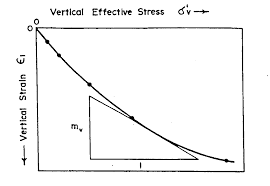The image above represents vertical strain.

To compute for vertical strain, five essential parameters are needed and these parameters are Vertical Stress (σv), Poisson’s Ratio (v), Principal Horizontal Stress Component 1 (σH1), Principal Horizontal Stress Component 2 (σH2) and Young’s Modulus (E).

The formula for calculating the vertical strain:

εv = v – vσH1 – vσH2) / E

Where:

εv = Vertical Strain
σv = Vertical Stress
v = Poisson’s Ratio
σH1 = Principal Horizontal Stress Component 1
σH2 = Principal Horizontal Stress Component 2
E = Young’s Modulus

Let’s solve an example;
Find the vertical strain with a vertical stress of 44, Poisson’s ratio of 5, principal horizontal stress component 1 of 6, principal horizontal stress component 2 of 4 and young’s modulus of 12.

This implies that;

σv = Vertical Stress = 44
v = Poisson’s Ratio = 5
σH1 = Principal Horizontal Stress Component 1 = 6
σH2 = Principal Horizontal Stress Component 2 = 4
E = Young’s Modulus = 12

εv = v – vσH1 – vσH2) / E
εv = (44 – 5(6) – 5(4)) / 12
εv = (44 – 30 – 20) / 12
εv = -6 / 12
εv = -0.5

Therefore, the vertical strain is -0.5.

Calculating the Vertical Stress when the Vertical Strain, Poisson’s Ratio, Principal Horizontal Stress Component 1, Principal Horizontal Stress Component 2 and Young Modulus is Given.

σv = (εv x E) + vσH1 + vσH2

Where:

σv = Vertical Stress
εv = Vertical Strain
v = Poisson’s Ratio
σH1 = Principal Horizontal Stress Component 1
σH2 = Principal Horizontal Stress Component 2
E = Young’s Modulus

Let’s solve an example;
Find the vertical stress with a vertical strain of 52, Poisson’s ratio of 12, principal horizontal stress component 1 of 15, principal horizontal stress component 2 of 10 and young’s modulus of 13.

This implies that;

εv = Vertical Strain = 52
v = Poisson’s Ratio = 12
σH1 = Principal Horizontal Stress Component 1 = 15
σH2 = Principal Horizontal Stress Component 2 = 10
E = Young’s Modulus = 13

σv = (εv x E) + vσH1 + vσH2
σv = (52 x 13) + 12(15) + 15(10)
σv = 676 + 180 + 150
σv = 1006

Therefore, the vertical stress is 1006.

Calculating the Poisson’s Ratio when the Vertical Strain, Vertical Stress, Principal Horizontal Stress Component 1, Principal Horizontal Stress Component 2 and Young Modulus is Given.

v = σv – (εv x E) / σH1 + σH2

Where:

v = Poisson’s Ratio
εv = Vertical Strain
σv = Vertical Stress
σH1 = Principal Horizontal Stress Component 1
σH2 = Principal Horizontal Stress Component 2
E = Young’s Modulus

Let’s solve an example;
Find the Poisson’s ratio with a vertical strain of 16, vertical stress of 110, principal horizontal stress component 1 of 7, principal horizontal stress component 2 of 13 and young’s modulus of 6.

This implies that;

εv = Vertical Strain = 16
σv = Vertical Stress = 110
σH1 = Principal Horizontal Stress Component 1 = 7
σH2 = Principal Horizontal Stress Component 2 = 13
E = Young’s Modulus = 6

v = σv – (εv x E) / σH1 + σH2
v = 110 – (16 x 6) / 7 + 13
v = 110 – 96 / 20
v = 14 / 20
v = 0.7

Therefore, the Poisson’s ratio is 0.7.

Calculating the Principal Horizontal Stress Component 1 when the Vertical Strain, Vertical Stress, Poisson’s Ratio, Principal Horizontal Stress Component 2 and Young Modulus is Given.

σH1 = -((Ev x E) – σv + vσH2 / v)

Where;

σH1 = Principal Horizontal Stress Component 1
εv = Vertical Strain
σv = Vertical Stress
v = Poisson’s Ratio
σH2 = Principal Horizontal Stress Component 2
E = Young’s Modulus

Let’s solve an example;
Find the principal horizontal stress component 1 with a vertical strain of 15, Poisson’s ratio of 10, vertical stress of 11, principal horizontal stress component 2 of 8 and young’s modulus of 14.

This implies that;

εv = Vertical Strain = 15
σv = Vertical Stress = 11
v = Poisson’s Ratio = 10
σH2 = Principal Horizontal Stress Component 2 = 8
E = Young’s Modulus = 14

σH1 = -((Ev x E) – σv + vσH2 / v)
σH1 = -((15 x 14) – 11 + 10(8) / 10)
σH1 = -(210 – 11 + 80 / 10)
σH1 = -(199 + 80 / 10)
σH1 = -(279 / 10)
σH1 = -(27.9)
σH1 = -27.9

Therefore, the principal horizontal stress component 1 is -27.9.

Calculating the Principal Horizontal Stress Component 2 when the Vertical Strain, Vertical Stress, Poisson’s Ratio, Principal Horizontal Stress Component 1 and Young Modulus is Given.

σH2 = -((Ev x E) – σv + vσH1 / v)

Where;

σH2 = Principal Horizontal Stress Component 2
εv = Vertical Strain
σv = Vertical Stress
v = Poisson’s Ratio
σH1 = Principal Horizontal Stress Component 1
E = Young’s Modulus

Let’s solve an example;
Find the principal horizontal stress component 2 with a vertical strain of 30, Poisson’s ratio of 18, vertical stress of 17, principal horizontal stress component 1 of 11 and young’s modulus of 19.

This implies that;

εv = Vertical Strain = 30
σv = Vertical Stress = 17
v = Poisson’s Ratio = 18
σH1 = Principal Horizontal Stress Component 1 = 11
E = Young’s Modulus = 19

σH2 = -((Ev x E) – σv + vσH1 / v)
σH2 = -((30 x 19) – 17 + 18(11) / 18)
σH2 = -(570 – 17 + 198 / 18)
σH2 = -(533 + 198 / 18)
σH2 = -(751 / 18)
σH2 = -(41.72)
σH2 = -41.72

Therefore, the principal horizontal stress component 2 is -41.72.

Calculating the Young Modulus when the Vertical Strain, Vertical Stress, Poisson’s Ratio, Principal Horizontal Stress Component 1 and Principal Horizontal Stress Component 2 is Given.

E = v – vσH1 – vσH2) / εv

Where;

E = Young’s Modulus
εv = Vertical Strain
σv = Vertical Stress
v = Poisson’s Ratio
σH1 = Principal Horizontal Stress Component 1
σH2 = Principal Horizontal Stress Component 2

Let’s solve an example;
Find the young’s modulus with a vertical strain of 20, vertical stress of 60, Poisson’s ratio of 2, principal horizontal stress component 1 of 7, principal horizontal stress component 2 of 9.

This implies that;

εv = Vertical Strain = 20
σv = Vertical Stress = 60
v = Poisson’s Ratio = 2
σH1 = Principal Horizontal Stress Component 1 = 7
σH2 = Principal Horizontal Stress Component 2 = 9

E = v – v(σH1) – v(σH2)) / εv
E = (60 – 2(7) – 2(9)) / 20
E = (60 – 14 – 18) / 20
E = (28) / 20
E = 1.4

Therefore, the young’s modulus is 1.4.

Nickzom Calculator – The Calculator Encyclopedia is capable of calculating the vertical strain.

To get the answer and workings of the vertical strain using the Nickzom Calculator – The Calculator Encyclopedia. First, you need to obtain the app.

You can get this app via any of these means:

To get access to the professional version via web, you need to register and subscribe for NGN 1,500 per annum to have utter access to all functionalities.
You can also try the demo version via https://www.nickzom.org/calculator

Apple (Paid) – https://itunes.apple.com/us/app/nickzom-calculator/id1331162702?mt=8
Once, you have obtained the calculator encyclopedia app, proceed to the Calculator Map, then click on Geology under Add-on.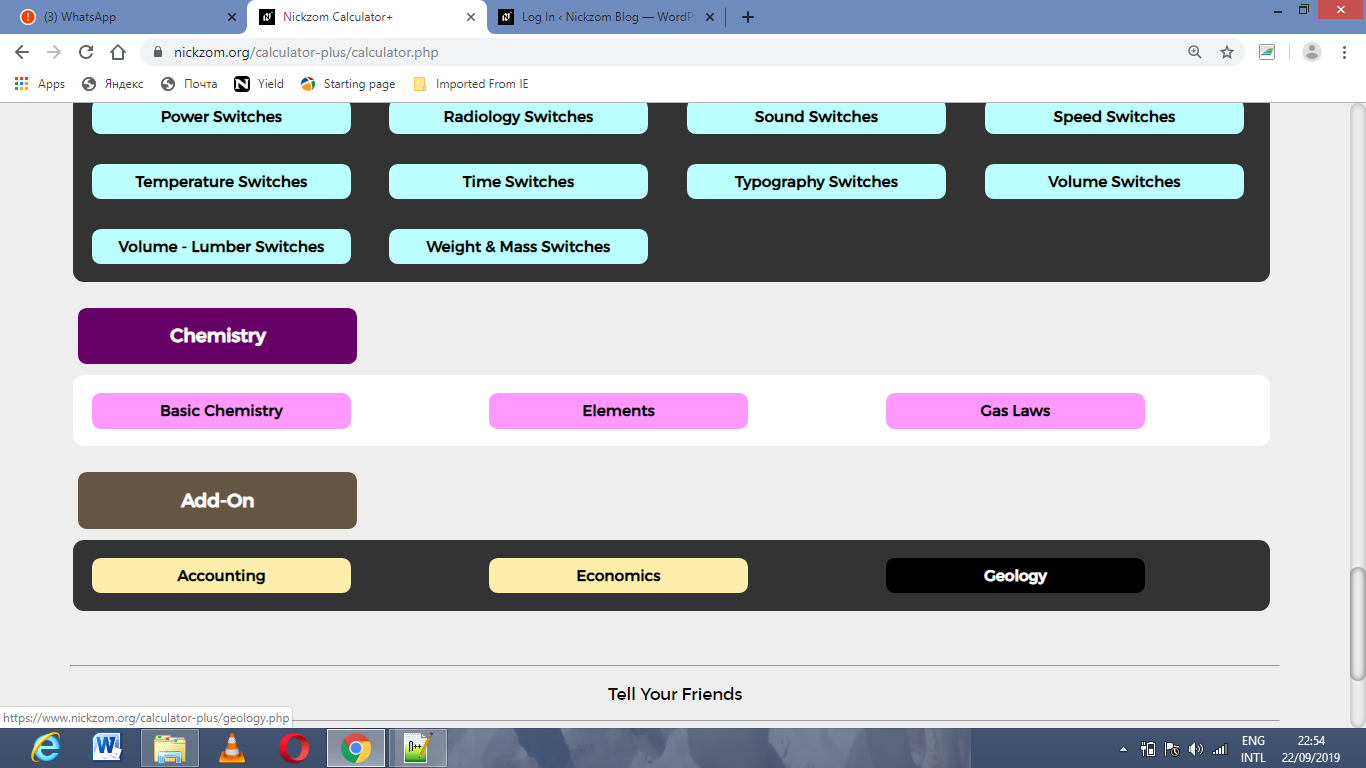Now, Click on Rock Mechanics under GeologyNow, Click on Vertical Strain under Rock MechanicsThe screenshot below displays the page or activity to enter your values, to get the answer for the vertical strain according to the respective parameters which are the Vertical Stress (σv), Poisson’s Ratio (v), Principal Horizontal Stress Component 1 (σH1), Principal Horizontal Stress Component 2 (σH2) and Young’s Modulus (E).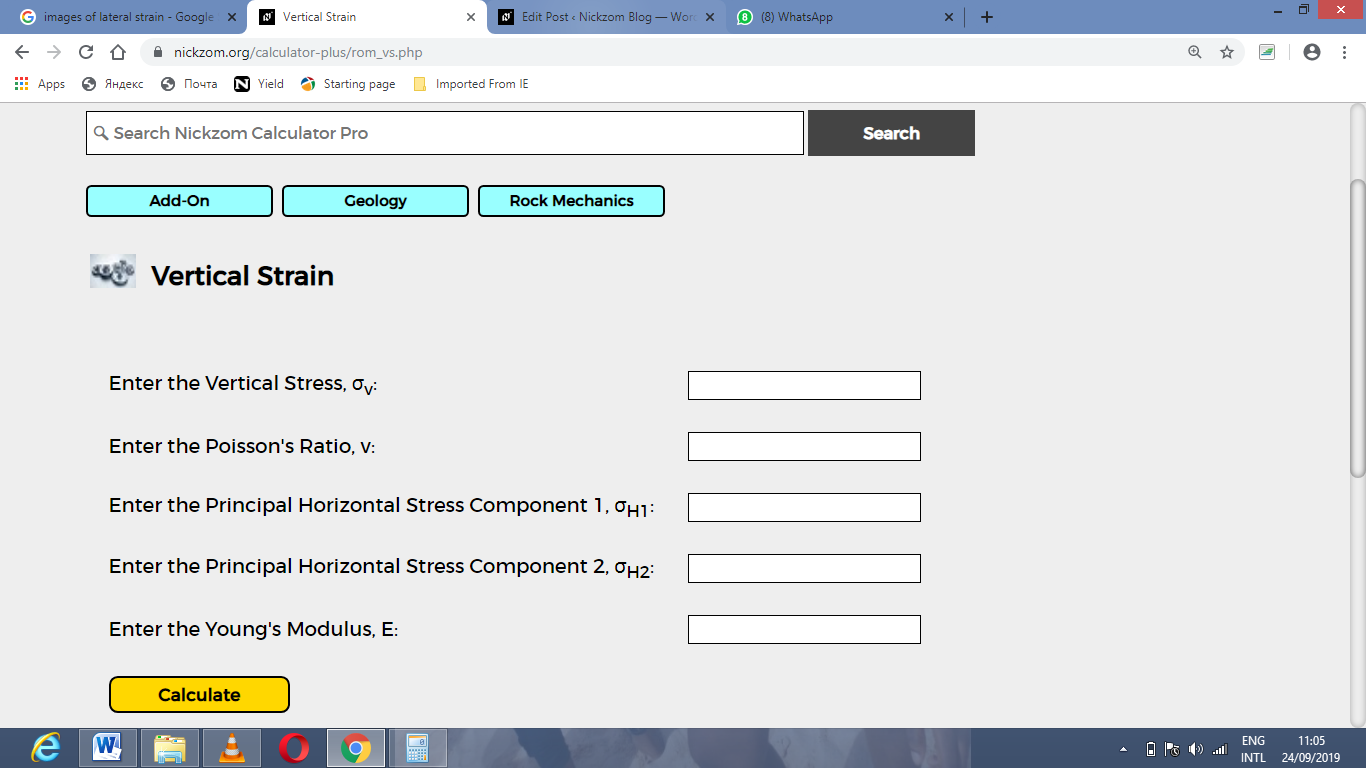Now, enter the values appropriately and accordingly for the parameters as required by the Vertical Stress (σv) is 44, Poisson’s Ratio (v) is 5, Principal Horizontal Stress Component 1 (σH1) is 6, Principal Horizontal Stress Component 2 (σH2) is 4 and Young’s Modulus (E) is 12.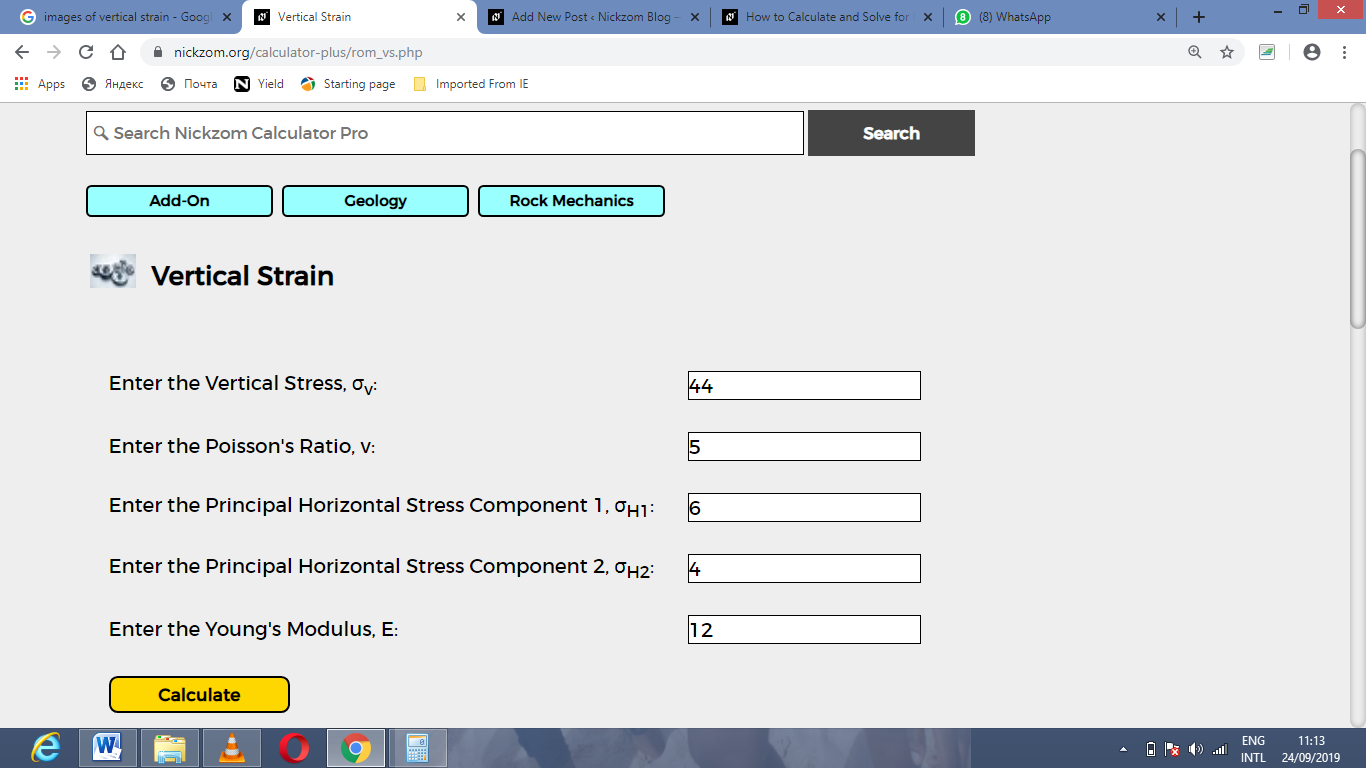Finally, Click on Calculate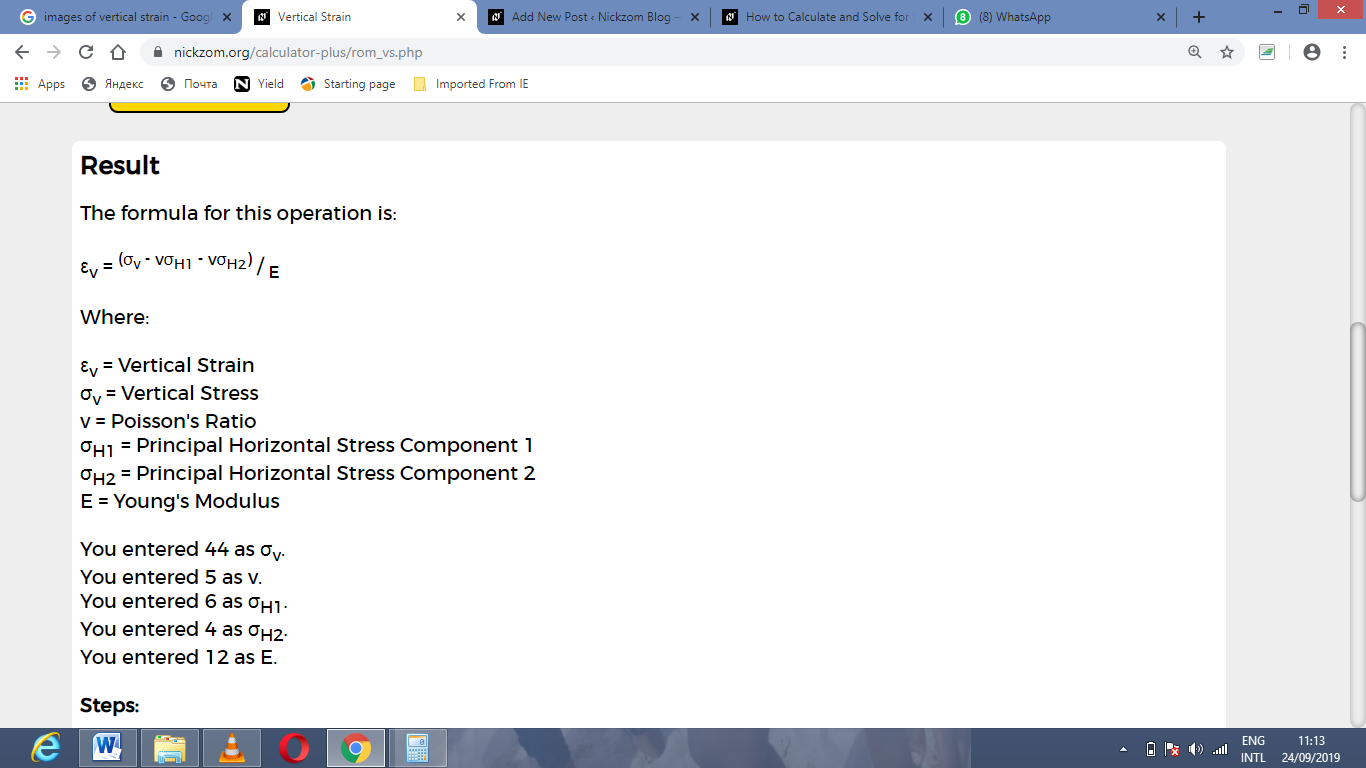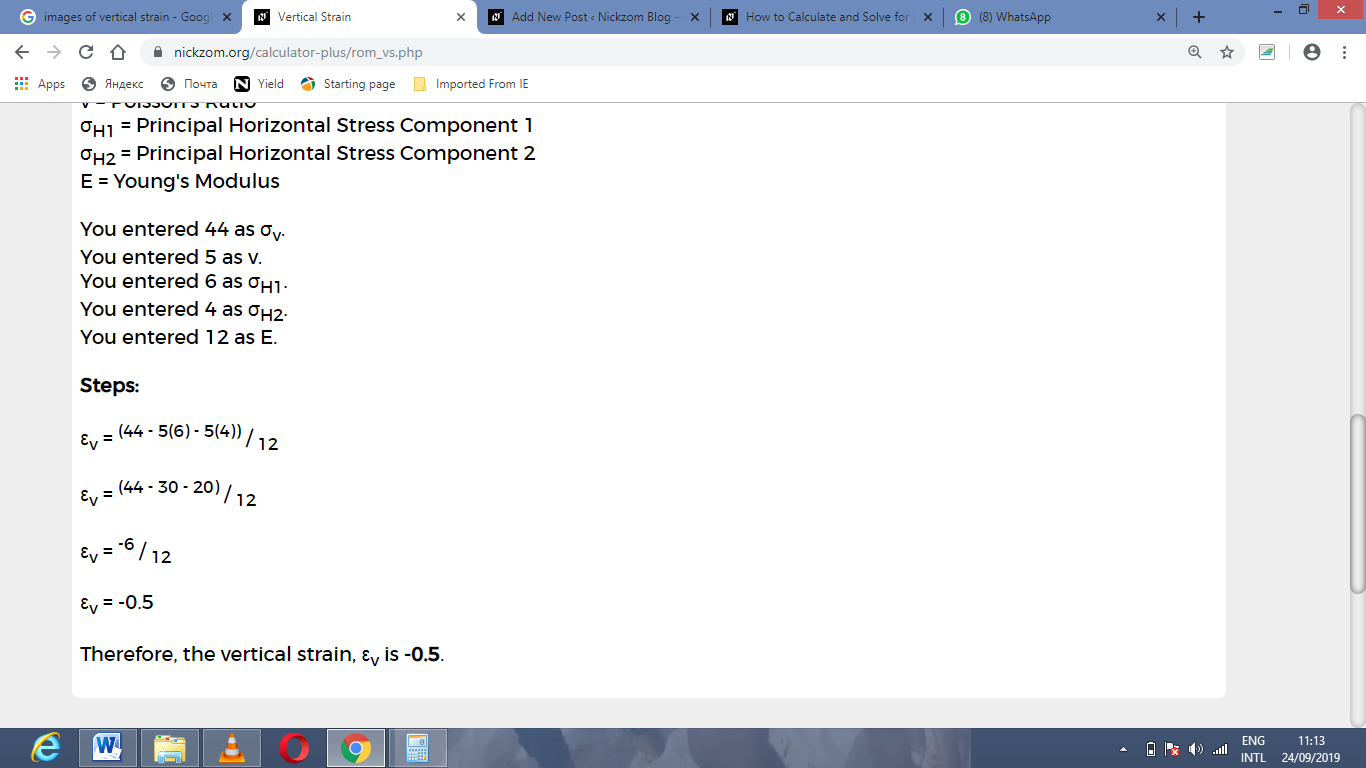As you can see from the screenshot above, Nickzom Calculator– The Calculator Encyclopedia solves for the vertical strain and presents the formula, workings and steps too.